1、rnn是什么

2、原理说明

2.1 rnn 和全连接神经网略的区别

2.2 RNN 简单图解释

2.3 RNN展开图解释

2.4 RNN的一些点

3、rnn的伪代码表示

4、来个小例子

5、rnn存在的问题

6、总结

## 1、rnn是什么

RNN 是循环神经网络的简称，他的英语是 Rerrent Neural Network = RNN，从命名中可以看到核心点是循环的神经网络，所以我们要理解循环的是什么？为什么要循环。这个会慢慢解释。不用着急。

RNN对具有序列特性的数据非常有效，它能挖掘数据中的时序信息以及语义信息，用人话解释就是RNN具有语境信息，可以理解上下文，在你进行分析的时候可以对全局进行考虑，可以用来挖掘数据之间的关系。

RNN 可以解决这个问题，RNN 会记录整个句子出现的信息，然后进行综合评判，最后才得出结论。

## 2、原理说明

### 2.1 rnn 和全连接神经网略的区别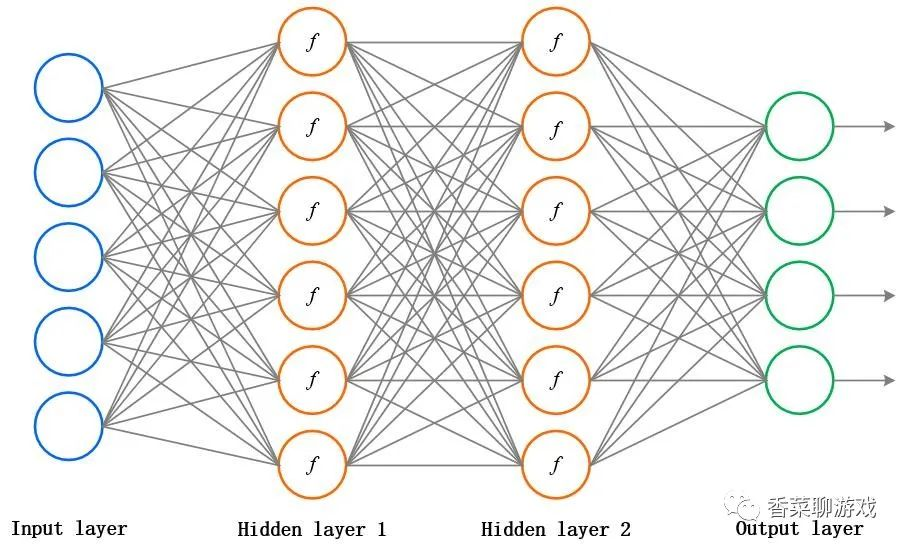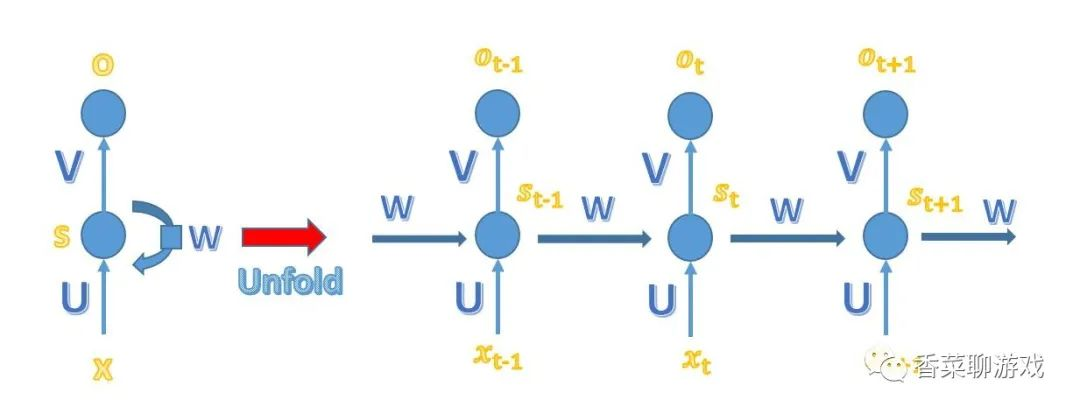### 2.2 RNN 简单图解释

x是一个向量，它表示输入层的值

U是输入层到隐藏层的权重矩阵

s是一个向量，它表示隐藏层的值

V是隐藏层到输出层的权重矩阵

o也是一个向量，它表示输出层的值``````def getHidenS(x,w,prevS):
return x * u + prevS*w
def getOutput(s):
return s * v``````

### 2.3 RNN展开图解释

o就是在输入每个单词时神经网络的输出，也就是说每次输入一个词向量的时候都会有一个输出，最后得出结论可以使用其中一个，或者综合考虑都可以，根据自己的需求

## 3、rnn的伪代码表示

``````w = 1 # 权重矩阵
u = 1 # 输入层到隐藏层的矩阵
v = 2 # 隐藏层到输出层的矩阵
prevS = 1 # 隐藏层的输出值

def getHidenS(x, w, prevS):
return x * u + prevS * w

def getOutput(s,v):
return s * v

sentance = [1,2,3]
for x in sentance:
prevS = getHidenS(x,u,prevS)
o = getOutput(prevS,v)
print('隐藏层的值：'+ str(prevS))
print('输出层的值 ：'+str(o))
print('----------------------')``````

prevS 保存了之前的记忆，每一次的输出都可以用来判断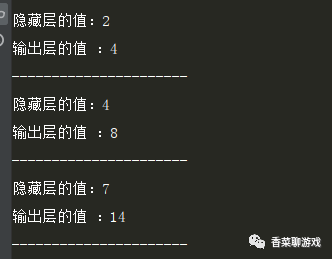## 4、来个小例子

``````import torch
from torch import nn
import numpy as np
import matplotlib.pyplot as plt

# https://www.cnblogs.com/lokvahkoor/p/12263953.html
# torch.manual_seed(1)    # reproducible

# 超参数定义
TIME_STEP = 10  # rnn time step
INPUT_SIZE = 1  # rnn input size
LR = 0.02  # 学习率
HIDDEN_SIZE = 32# 隐藏层神经元个数
EPOCH = 100

# 横向坐标，产出100个float点
steps = np.linspace(0, np.pi * 2, 100, dtype=np.float32)  # float32 for converting torch FloatTensor
x_np = np.sin(steps)
y_np = np.cos(steps)
# 输入的参数是sin的序列，输出是余弦序列
plt.plot(steps, y_np, 'r-', label='target (cos)')
plt.plot(steps, x_np, 'b-', label='input (sin)')
plt.legend(loc='best')
plt.show()

input("请回车：")

class RNN(nn.Module):
def __init__(self):
super(RNN, self).__init__()

self.rnn = nn.RNN(
input_size=INPUT_SIZE,
hidden_size=HIDDEN_SIZE,  # 隐藏神经元的数量
num_layers=1,  # 一层rnn
batch_first=True,  # input & output will has batch size as 1s dimension. e.g. (batch, time_step, input_size)
)
self.out = nn.Linear(HIDDEN_SIZE, 1)

def forward(self, x, h_state):
# x = (batch, time_step, input_size)
# h_state = (n_layers, batch, hidden_size)
# r_out = (batch, time_step, hidden_size)

out, h_state = self.rnn(x, h_state)

out = out.view(-1, HIDDEN_SIZE) #(10,32)
out = self.out(out) #(10,1)
out = out.unsqueeze(dim=0) # (1,10,1) -> (n_layers, batch, hidden_size)
return out, h_state

rnn = RNN()
print(rnn)

optimizer = torch.optim.Adam(rnn.parameters(), lr=LR)  # 优化器
loss_func = nn.MSELoss()  # 损失函数

h_state = None  # 隐藏层的输出值

plt.figure(1, figsize=(12, 5))
plt.ion()  # continuously plot

for step in range(EPOCH):
# 每次生成新的数据，整体的趋势是拟合成cos函数曲线
start, end = step * np.pi, (step + 1) * np.pi  # time range
# use sin predicts cos
steps = np.linspace(start, end, TIME_STEP, dtype=np.float32,
endpoint=False)  # float32 for converting torch FloatTensor
x_np = np.sin(steps)
y_np = np.cos(steps)
# np.newaxis的功能:插入新维度,(1,10,1)
# shape (batch, time_step, input_size)
# 表示每一批送进去一个
x = torch.from_numpy(x_np[np.newaxis, :, np.newaxis])
y = torch.from_numpy(y_np[np.newaxis, :, np.newaxis])

prediction, h_state = rnn(x, h_state)  # 计算输出
# 将上一步的隐藏层的结果进行保存，在下次输入
h_state = h_state.data  # repack the hidden state, break the connection from last iteration

loss = loss_func(prediction, y)  # 计算误差
loss.backward()  # 反向传播
optimizer.step()  # 优化参数

# 开始画图
plt.plot(steps, y_np.flatten(), 'r-')
plt.plot(steps, prediction.data.numpy().flatten(), 'b-')
plt.draw()
plt.pause(0.05)

plt.ioff()
plt.show()``````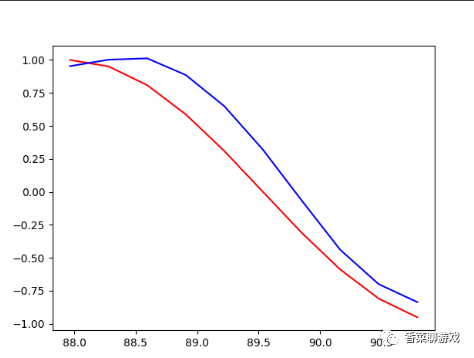## 6、总结

RNN的关键点是记忆功能，也就是保存了语境信息，但是也存在一些问题，后面我们会分析怎么解决这个问题。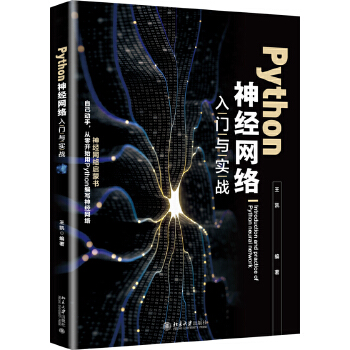《Python神经网络入门与实战》(王凯)【摘要 书评 试读】- 京东图书

《Python神经网络入门与实战》(王凯)【简介_书评_在线阅读】 - 当当图书